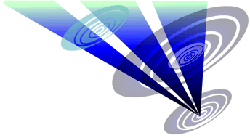Reconstruction of the 2019 Preston Candover formation 1.Draw a circle. Draw and extend the horizontal and vertical centerlines. 2.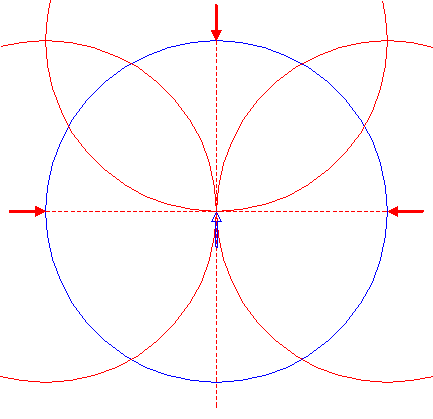Copy circle 1 three times, to its righthand, upper, and lefthand intersections with the horizontal and vertical centerlines. 3.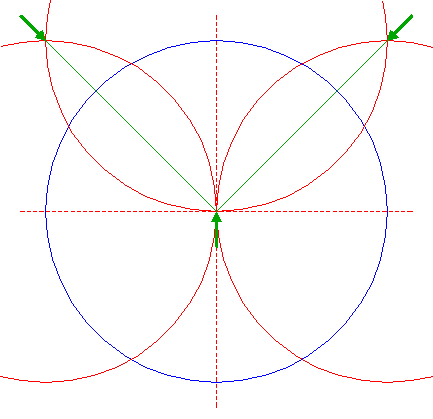Draw the two connecting lines between the intersections of the upper and righthand, and between the intersections of the upper and lefthand circles 2. 4.Draw the connecting line between the righthand intersection of circle 1 and the horizontal centerline, and its upper intersection with the vertical centerline. 5.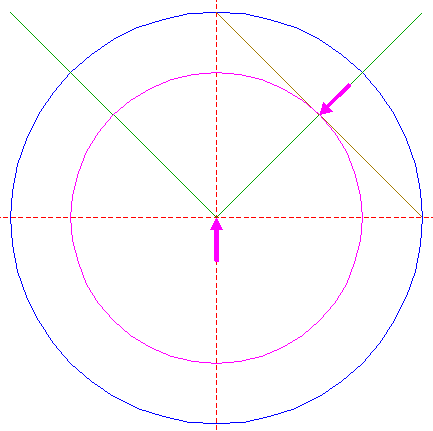Construct a circle concentric to circle 1, tangent to line 4. 6.Construct the inscribed square of circle 5, with one angular point on the righthand line 3. 7.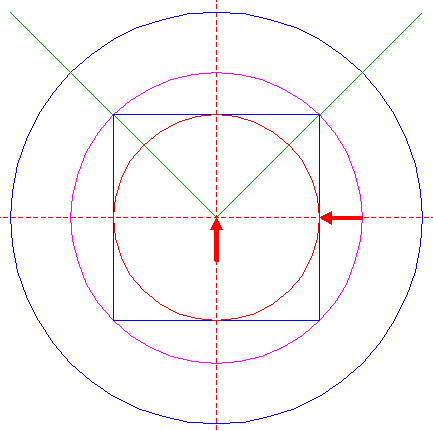Construct the inscribed circle of square 6. 8.Construct the inscribed dodecagon (regular 12-sided polygon) of circle 7, pointing to the right. 9.Construct the inscribed circle of dodecagon 8. 10.Construct the inscribed equilateral triangle of circle 9, pointing up. 11.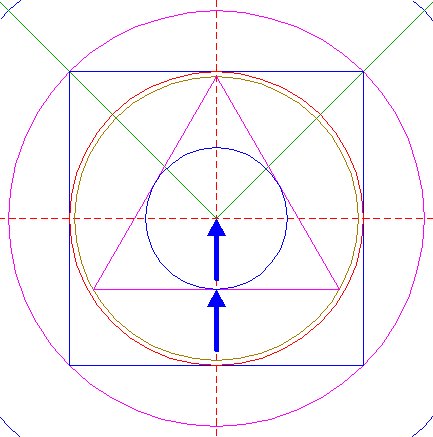Construct the inscribed circle of triangle 10. 12.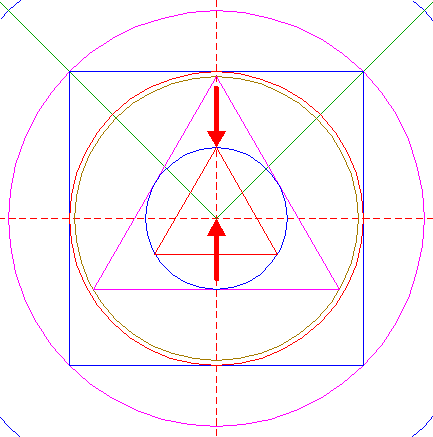Construct the inscribed equilateral triangle of circle 11, pointing up. 13.Extend the sides of triangle 12 in both directions, upto triangle 10. 14.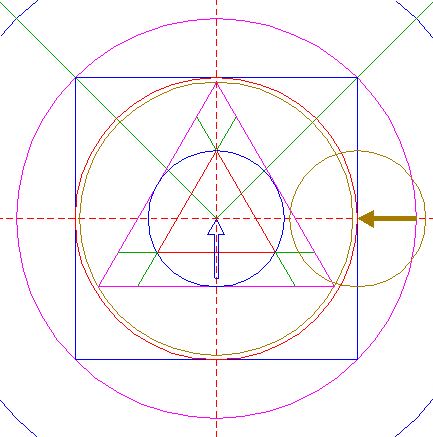Copy circle 11 to the righthand intersection of circle 7 and the horizontal centerline. 15.Construct a circle concentric to circle 1, tangent to circle 15 at the righthand side. 16.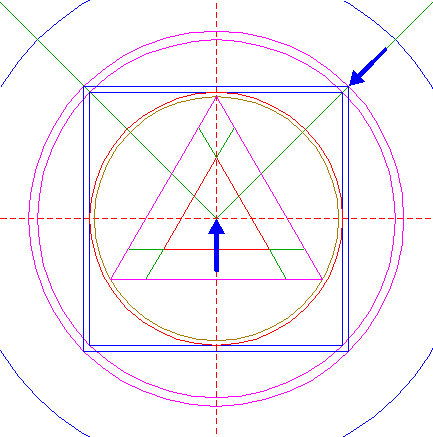Construct the inscribed square of circle 15, with one angular point on the righthand line 3. 17.Construct the inscribed circle of square 16. 18.Construct the inscribed equilateral triangle of circle 17, pointing up. 19.Construct a circle centered at the upper righthand angular point of square 6, passing through the upper righthand angular point of square 16. 20.Construct a circle concentric to circle 1, tangent to circle 19 at the lower lefthand side. 21.Copy circle 19 to the righthand intersection of circle 9 and the horizontal centerline. 22.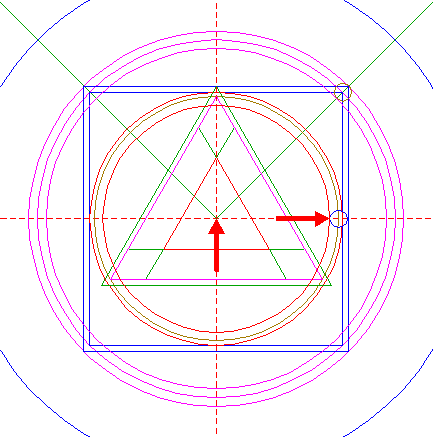Construct a circle concentric to circle 1, tangent to circle 21 at the lefthand side. 23.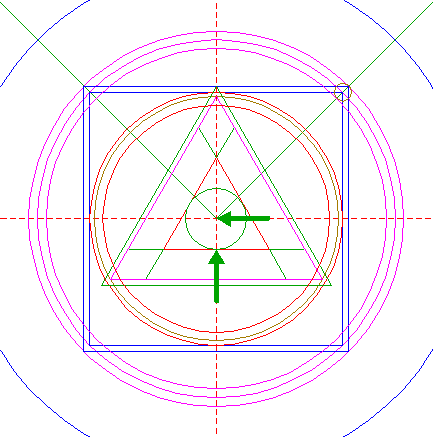Construct the inscribed circle of triangle 12. 24.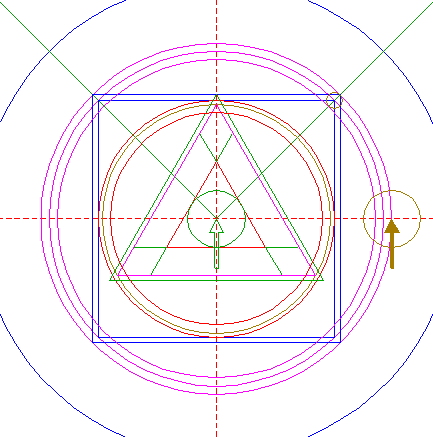Copy circle 23 to the righthand intersection of circle 15 and the horizontal centerline. 25.Construct a circle concentric to circle 1, tangent to circle 24 at the righthand side. 26.Copy circle 23 to the righthand intersection of circle 25 and the horizontal centerline, and move this circle (copy and delete original) to its own corresponding intersection. 27.Construct a circle concentric to circle 1, tangent to circle 26 at the righthand side. 28.Construct the circumscribed dodecagon of circle 27, with one angular point on the righthand line 3. 29.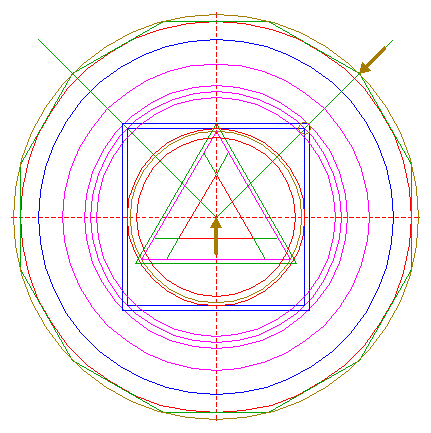Construct the circumscribed circle of dodecagon 28. 30.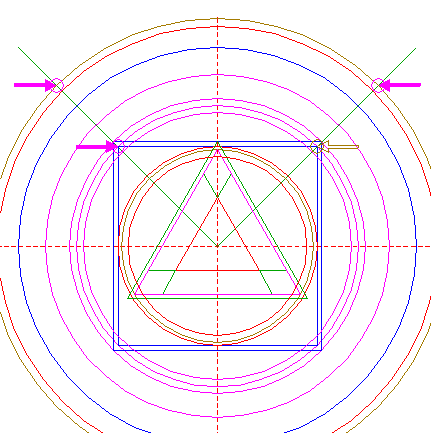Copy circle 19 three times, to the upper lefthand angular point of square 6, and to the intersections of circle 29 and lines 3. 31.Construct two lines, parallel to lines 3, tangent to circles 19 and 30 at the lower righthand and lower lefthand sides, respectively. 32.Circles 1, 5, 9, 15, 20, 22, 25, 27, and 29, squares 6, and 16, triangles 10, 12, and 18, and lines 3, 13, and 31, are used for the final reconstruction. 33.Remove all parts not visible within the formation itself. 34.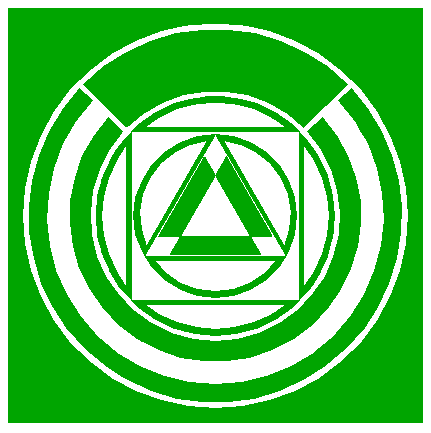Colour all areas corresponding to standing... 35.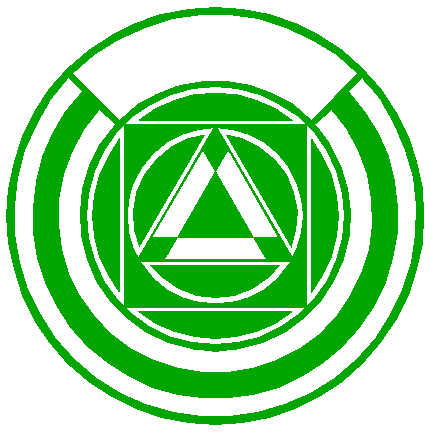...or to flattened crop, and finish the reconstruction of the 2019 Preston Candover formation. 36.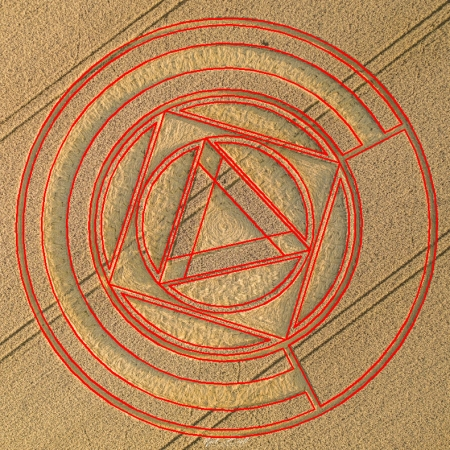The final result, matched with two aerial images.